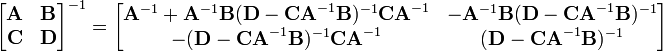### Nexus for Matrix Inversion

I have needed matrix inversion logic on several projects and in several computer languages. This is an attempt at cross-fertilization.

In Algol 68 there is this which is a plain inversion routine. There is this more exotic routine based on decomposing the matrix into blocks:copied from here. The first of these inverts all invertible matrices. The 2nd, as it stands, certainly does not. There are probably ill-conditioned matrices that other routines could handle better.

The inversion routine in this Scheme matrix suite inverts all invertible matrices M and returns a non-zero vector x such that Mx=0 as it reports a singular matrix—a constructive proof of its singularity and a value sought in some eigenvector algorithms. The code can work with exact rational complex numbers, or even finite fields. Some of the logic is described here.

Some C code with calling logic included. It unifies solution and inversion.

This OCaml module includes matrix inversion code.

Scheme code for Inverting Matrices in Arbitrary Fields
Algol 68 that unifies Solution and Inversion
Scheme repository module with inversion
Self-contained Scheme code